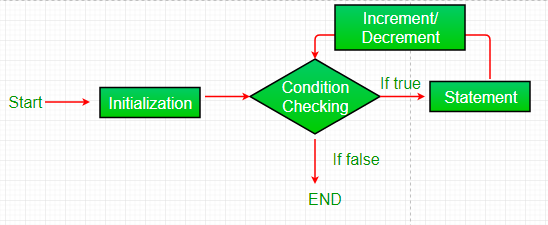Open In App

# Understanding for loops in Java

Suppose it is required to print numbers from 1 to 5. One possible way to do this is with the help of below code:

 `class` `GFG {``    ``public` `static` `void` `main(String args[])``    ``{`` ` `        ``int` `a;``        ``a = ``1``;``        ``System.out.println(a);``        ``a=``2``;``        ``System.out.println(a);``        ``a=``3``;``        ``System.out.println(a);``        ``a=``4``;``        ``System.out.println(a);``        ``a=``5``;``        ``System.out.println(a);``    ``}``}`

The above code might look simple, but it has many disadvantages, such as:

• Fixed Code: The code is made to print only numbers 1 to 5. What if some other numbers or some other pattern is required? In that case, this solution would suggest to write another program with the new required values or change each individual values in the existing program. And this will go on every time resulting in a long and fixed structure written multiple times.
• Repetitive Code: As mentioned in the last point, this solution suggests to write the whole program again with the modified value or modify the values in the existing program every time. But what if 100s of such patterns are required. This would result in a lot of repetitive and unnecessary code.
• Not Generalised: The suggested solution is not generalised. It means that the numbers to be printed are fed to the code by the user statically. There is no predefined pattern that the code is following and printing the code according to the pattern.
• Not Scalable: Every code written in computer science must be written in a scalable manner. It means that the code must run fine with minimum number of changes to print the numbers 1 to 100.

Here comes the “Loop” for help, whenever repetition of a process is required.

### What are Loops?

Looping in programming languages is a feature which facilitates the execution of a set of instructions/functions repeatedly while some condition evaluates to true.
Java provides three ways for executing the loops. While all the ways provide similar basic functionality, they differ in their syntax and condition checking time.

1. while loop: A while loop is a control flow statement that allows code to be executed repeatedly based on a given Boolean condition. The while loop can be thought of as a repeating if statement.
Syntax :

```while (boolean condition)
{
loop statements...
}
```
2. for loop: for loop provides a concise way of writing the loop structure. Unlike a while loop, a for statement consumes the initialization, condition and increment/decrement in one line thereby providing a shorter, easy to debug structure of looping.
Syntax:

```for (initialization condition; testing condition;
increment/decrement)
{
statement(s)
}
```

Flowchart:Enhanced For loop

Java also includes another version of for loop introduced in Java 5. Enhanced for loop provides a simpler way to iterate through the elements of a collection or array. It is inflexible and should be used only when there is a need to iterate through the elements in sequential manner without knowing the index of currently processed element.
Syntax:

```for (T element:Collection obj/array)
{
statement(s)
}
```
3. do while: do while loop is similar to while loop with only difference that it checks for condition after executing the statements, and therefore is an example of Exit Control Loop.
Syntax:

```do
{
statements..
}
while (condition);
```
4. ### How to write a loop?

On carefully observing the code given above, it can be broken into following processes or steps:
1) Initialize a value to a variable
2) Print the value
3) Change the value
4) Print the value

These processes are repeated again and again. This can be replaced with a loop as follows:

• In the code shown above, initialization is a=1, the same thing can be made in for loop.
• The second expression is condition. The condition should specify how many times the loop should execute. It depends on the number of times the process should be repeated. In this case, it should run for 5 times. The value of “a” starts from 1 and it should be printed till its value is 5. So the condition to be added is a<=5.
• The third expression is the updation. Every time the loop variable, here ‘a’, needs to be updated according to the expected code. Here the value of ‘a’ is incremented by 1 on every print operation. Hence a++ would be suitable in this situation.
• The last expression is the body, which is the required action to be performed repetitively. Here the value of ‘a’ is printed on every execution.

Below is the execution of the required code with the help of Loop:

 `class` `GFG {``    ``public` `static` `void` `main(String args[])``    ``{``        ``int` `a;``        ``for` `(a = ``1``; a <= ``5``; a++) {``            ``System.out.println(a);``        ``}``    ``}``}`